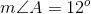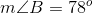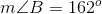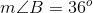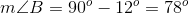GED Math : Complementary Angles

Example Questions

Example Question #41 : Angle Geometry

The image is not to scale.

If the sum of two angles results in a complementary angle, what is the measure of the unknown angle?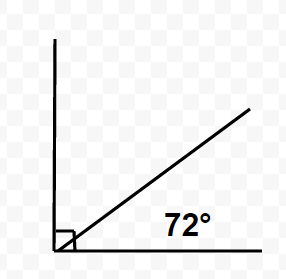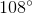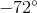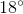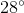Explanation:

With the provided image, we are asked to solve for the measure of the unknown angle.First, we must understand some information before attempting to solve the problem. The problem provides the information that the two angles summed up result in a complimentary angle. This is another way to say that when we add the measures of the two angles, it will equal.

This becomes a problem where we solve for a missing variable now. We can call the unknown angle x. We would set this up in equation format accordingly: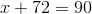Now, we can solve for x.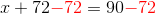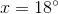Therefore, the unknown angle is.

Example Question #42 : Complementary Angles

The image is not to scale.

If the sum of two angles results in a complementary angle, what is the measure of the unknown angle?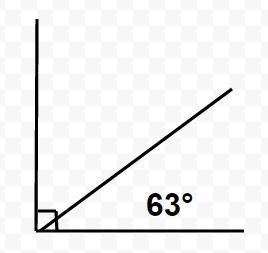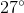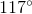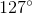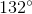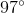Explanation:

With the provided image, we are asked to solve for the measure of the unknown angle.First, we must understand some information before attempting to solve the problem. The problem provides the information that the two angles summed up result in a complimentary angle. This is another way to say that when we add the measures of the two angles, it will equal.

This becomes a problem where we solve for a missing variable now. We can call the unknown angle x. We would set this up in equation format accordingly: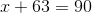Now, we can solve for x.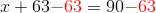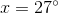Therefore, the unknown angle is.

Example Question #43 : Complementary Angles

Which of the following angles forms a complementary angle pair with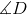?

Given that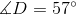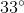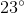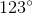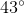Explanation:

Which of the following angles forms a complementary angle pair with?

Given thatComplementary angles sum up to 90 degrees. Therefore, to find our answer, we simply need to do the following: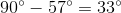So, our answer is 33 degrees

Example Question #44 : Complementary Angles

Find the the measure of angle B if it is complement of angle A: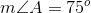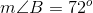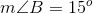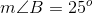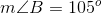Explanation:

If two angles are complementary, that means the sum of their degrees of measure will add up to 90. In order to find the measure of angle B, subtract angle A from 90 like shown: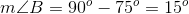This gives us a final answer of 15 degrees for angle B.

Example Question #45 : Complementary Angles

Find the the measure of angle B if it is complement of angle A: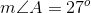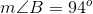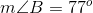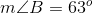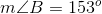Explanation:

If two angles are complementary, that means the sum of their degrees of measure will add up to 90. In order to find the measure of angle B, subtract angle A from 90 like shown: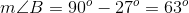This gives us a final answer of 63 degrees for angle B.

Example Question #46 : Complementary Angles

Find the the measure of angle B if it is complement of angle A: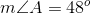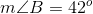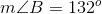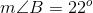Explanation:

If two angles are complementary, that means the sum of their degrees of measure will add up to 90. In order to find the measure of angle B, subtract angle A from 90 like shown:This gives us a final answer of 42 degrees for angle B.

Example Question #47 : Complementary Angles

Find the the measure of angle B if it is complement of angle A: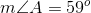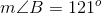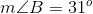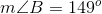Explanation:

If two angles are complementary, that means the sum of their degrees of measure will add up to 90. In order to find the measure of angle B, subtract angle A from 90 like shown: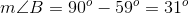This gives us a final answer of 31 degrees for angle B.

Example Question #48 : Complementary Angles

Find the the measure of angle B if it is complement of angle A: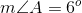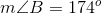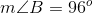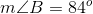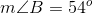Explanation:

If two angles are complementary, that means the sum of their degrees of measure will add up to 90. In order to find the measure of angle B, subtract angle A from 90 like shown: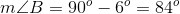This gives us a final answer of 84 degrees for angle B.

Example Question #49 : Complementary Angles

Find the the measure of angle B if it is complement of angle A: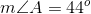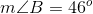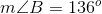Explanation:

If two angles are complementary, that means the sum of their degrees of measure will add up to 90. In order to find the measure of angle B, subtract angle A from 90 like shown: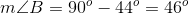This gives us a final answer of 46 degrees for angle B.

Example Question #50 : Complementary Angles

Find the the measure of angle B if it is complement of angle A: